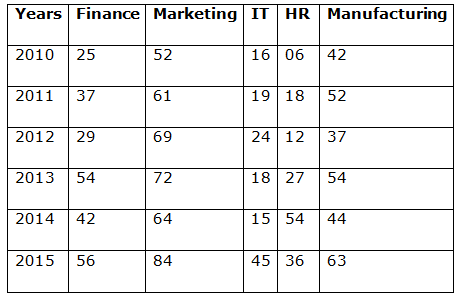# NIACL AO Prelims – Quantitative Aptitude Questions Day- 87

Dear Readers, Bank Exam Race for the Year 2019 is already started, To enrich your preparation here we have providing new series of Practice Questions on Quantitative Aptitude – Section. Candidates those who are preparing for NIACL AO Prelims 2019 Exams can practice these questions daily and make your preparation effective.

[WpProQuiz 5017]

1) Applied to a bill for Rs. 2,00,000, the difference between a discount of 40 % and two successive discount of 36 % and 4 % is?

a) 3640

b) 4820

c) 5260

d) 2880

e) None of these

2) The total area of a circle and rectangle is 991 Sq cm. The radius of the circle is 14 cm. What is the sum of the circumference of circle and perimeter of rectangle, if length of the rectangle is 25 cm?

a) 124 cm

b) 168 cm

c) 156 cm

d) 142 cm

e) None of these

3) Sree and Subha entered into a partnership with an investment of Rs. 30000 and 40000 respectively. At the end of 4 monthly Sree withdraw 10000 and Subha invested Rs. 10000 more. Find the share of Subha, if the total profit at the end of the year is Rs. 36000.

a) 12000

b) 10000

c) 24000

d) 16000

e) None of these

4) Ravi spent 40% of his salary on food, 25% of the remaining on clothes, 35% of the remaining on miscellaneous expenditure. If he saves Rs. 58500, find the average amount he spent on food and clothes?

a) 45000

b) 55000

c) 44000

d) 35000

e) None of these

5) A bag contains 4 red marbles, 5 yellow marbles and 6 pink marbles. If three marbles are taken at random, than what is the probability that 2 marbles are pink?

a) 27/91

b) 15/91

c) 36/49

d) 13/49

e) None of these

Directions (6 – 10): Study the following table carefully to answer the questions that follow:

Number of employees retired from a company ABC during 6 different years.6) What is the difference between the total number of employees retired from Finance Dept in the year 2012, 2013 and 2014 and the number of employees retired from marketing dept in the year 2014?

a) 47

b) 64

c) 36

d) 61

e) 68

7) Total number of employees retired from Manufacturing in the years 2011 and 2012 together was approximately what per cent of the total number of employees retired from IT over all the years together?

a) 70%

b) 54%

c) 65%

d) 75%

e) 80%

8) What was the average number of employees retired together in the year 2013?

a) 43

b) 45

c) 23

d) 52

e) 54

9) In which department the number of the employees retired continuously increased from the year 2010 to 2015?

a) Finance

b) Marketing and Manufacturing only

c) HR

d) IT only

e) None of these

10) What was the respective ratio between the number of employees retired from Marketing in the year 2013 and number of employees retired from HR Dept in the year 2014?

a) 3: 5

b) 4: 3

c) 3: 7

d) 13: 3

e) 7:2

200000*(60/100) = 120000

200000*(64/100)*(96/100) = 122880

The difference is,

= > 122880 – 120000

= >2880

Πr2 + lb = 991

(22/7)*14*14 + 25b = 991

616 + 25b = 991

25b = 991 – 616

25b = 375

=>b = 15 cm

Circumference of circle = 2πr = 2*(22/7)*14 = 88 cm

Perimeter of rectangle = 2(l + b) = 2(25 + 15)

= > 2 * 40 = 80 cm

Required answer = 88 + 80 = 168 cm

Ratio of share of Sree and Subha is,

= > [30000*4 + 20000*8]: [40000*4 + 50000*8]

= > 280000: 560000

= > 1: 2

Total profit = Rs. 36000

3’s = 36000

1’s = 12000

Share of Subha = 2’s = Rs. 24000

Salary*(60/100)*(75/100)*(65/100) = 58500

Salary = 58500*(100/60)*(100/75)*(100/65) = 200000

Spending on food = 200000 * (40/100) = 80000

Spending on clothes = 200000 * (60/100) * (25/100) = 30000

Sum of spending on food and cloth together = 80000 + 30000 = 110000

Average = 110000/2 = Rs. 55000

Total probability = 15C3

Required probability = 6C2 and 9C1

The probability that 2 marbles are pink,

= > [6C2 and 9C1] / 15C3­

= > (15*9) / (5*7*13)

= > 27/91

Direction (6-10) :

Number of employees retired from finance dept in the years 2012, 2013 and 2014

= (29+54+42) =125

Number of employees retired from marketing dept in the year 2014 = 64

Required difference = (125 – 64) = 61

Total number of soldiers retired from IT over all the years together.

= (16 + 19 + 24 + 18 + 15 + 45) = 137

Number of employees retired from Manufacturing in the years 2011 and 2012

= (37+52) = 89

Required percentage = (89/137) x 100 = 65%

Required average

= (54+72+18+27+54)/5

= 225/5

= 45

It is obvious from the table.

In no department, the number of employees retired continuously increased from the year 2010 to 2015

The number of employees retired from marketing dept in 2013 = 72

The number of employees retired from HR dept in the year 2014 = 54

Required ratio = 72: 54 = 4: 3